## 7.2.1 Problem Formulation

A state space is defined that considers the configurations of all robots simultaneously,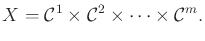(7.6)

A state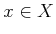specifies all robot configurations and may be expressed as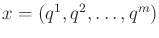. The dimension ofis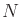, which is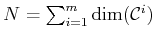.

There are two sources of obstacle regions in the state space: 1) robot-obstacle collisions, and 2) robot-robot collisions. For eachsuch that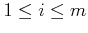, the subset ofthat corresponds to robot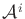in collision with the obstacle region,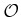, is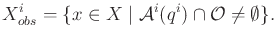(7.7)

This only models the robot-obstacle collisions.

For each pair,and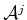, of robots, the subset ofthat corresponds toin collision withis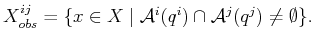(7.8)

Both (7.7) and (7.8) will be combined in (7.10) later to yield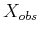.

Formulation 7..2 (Multiple-Robot Motion Planning)
1. The world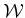and obstacle regionare the same as in Formulation 4.1.
2. There are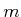robots,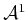,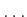,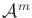, each of which may consist of one or more bodies.
3. Each robot, forfromto, has an associated configuration space,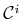.
4. The state spaceis defined as the Cartesian product(7.9)

The obstacle region inis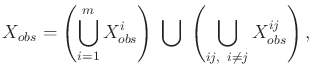(7.10)

in which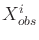and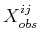are the robot-obstacle and robot-robot collision states from (7.7) and (7.8), respectively.
5. A state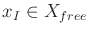is designated as the initial state, in which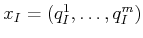. For eachsuch that,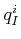specifies the initial configuration of.
6. A state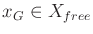is designated as the goal state, in which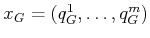.
7. The task is to compute a continuous path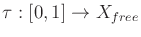such that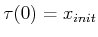and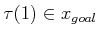.

Subsections
Steven M LaValle 2020-08-14